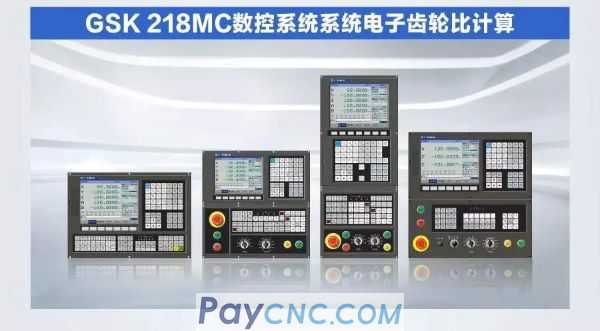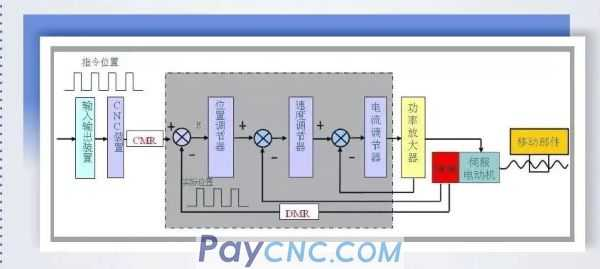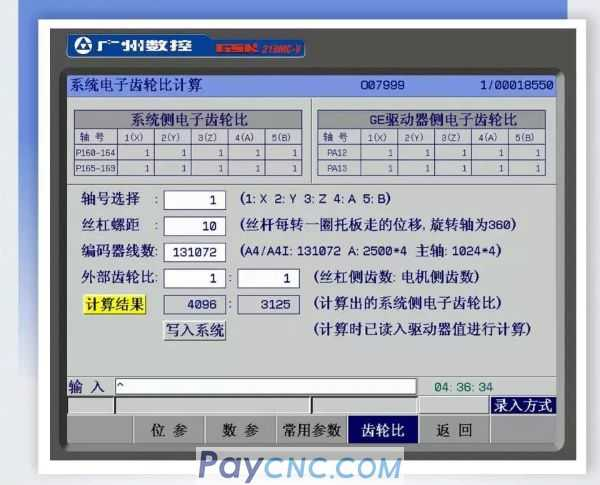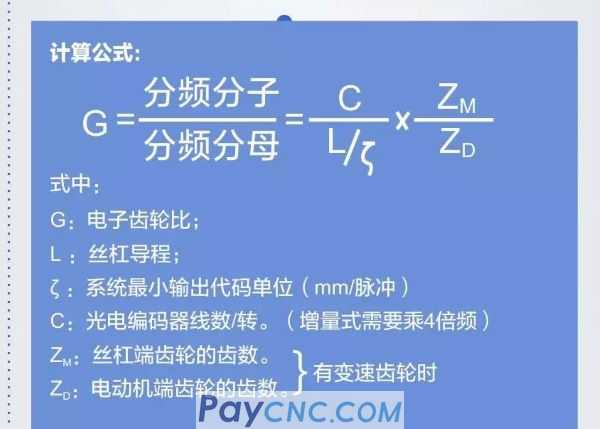01 In order to control the movement path of the tool, the CNC system must differentiate the continuous movement of the coordinate axis into the movement of a unit pulse. The actual movement corresponding to this unit pulse is called the minimum movement increment of the position control system or the command pulse equivalent, Command unit, usually 0.001mm. After the coordinate axis enters position control, the CNC outputs command pulses according to the minimum movement increment, and the actual position is given feedback pulses by the position detection device (such as pulse encoder, PulseCoder) according to its resolution. The displacement amount corresponding to one feedback pulse is called the detection pulse equivalent or detection unit.When the movement distance of the machine tool is inconsistent with the displacement distance displayed by the system coordinates, the system parameters P160~P164 and P165~P169 can be modified to adjust the electronic gear ratio to adapt to different mechanical transmission ratios. Set the position code pulse frequency multiplication (electronic gear). In the position control mode, through the setting of parameters, it can be easily matched with various pulse sources to achieve the ideal control resolution (ie angle/pulse) of the knife user. The system electronic gear ratio calculation of the 218MC system provides customers with a convenient, concise and intuitive operation interface. The system electronic gear ratio calculation is introduced in detail below:System side: Frequency division numerator: system parameters NO.160, NO.161, NO.162, NO.163, NO.164 (code frequency multiplication factor) Frequency division denominator: system parameters NO.165, NO.166, NO.167, NO.168, NO.169 (code frequency multiplication factor) Digital servo side: Frequency division numerator: parameter PA12 (code frequency multiplication factor) Frequency division denominator: parameter PA13 (code frequency multiplication factor)           02 Use the system electronic gear ratio calculation interface Select the corresponding axis number; Set the screw pitch of the corresponding axis; (the rotation axis is set to 360) Enter the number of motor encoder lines; (A6: 8388608 A4: 131072) When there is a transmission gear, input the number of teeth of the screw end gear: the number of teeth of the motor end gearNote: This interface is convenient for users to calculate the electronic gear ratio of the system. By default, the electronic gear ratio on the drive unit side needs to be kept at 1:1 (note that the bit parameter NO: 5#1 minimum moving unit), and the calculation result can be written into the system side. .           03 Conclusion Through the above instructions, you can quickly understand the electronic gear ratio calculation of the system, and you can also use the electronic gear ratio calculation interface of the system. This interface effectively provides an intuitive and concise display interface, which makes the customer familiar with the operation and the convenience during the debugging process. Ensure the stability of processing and debugging.